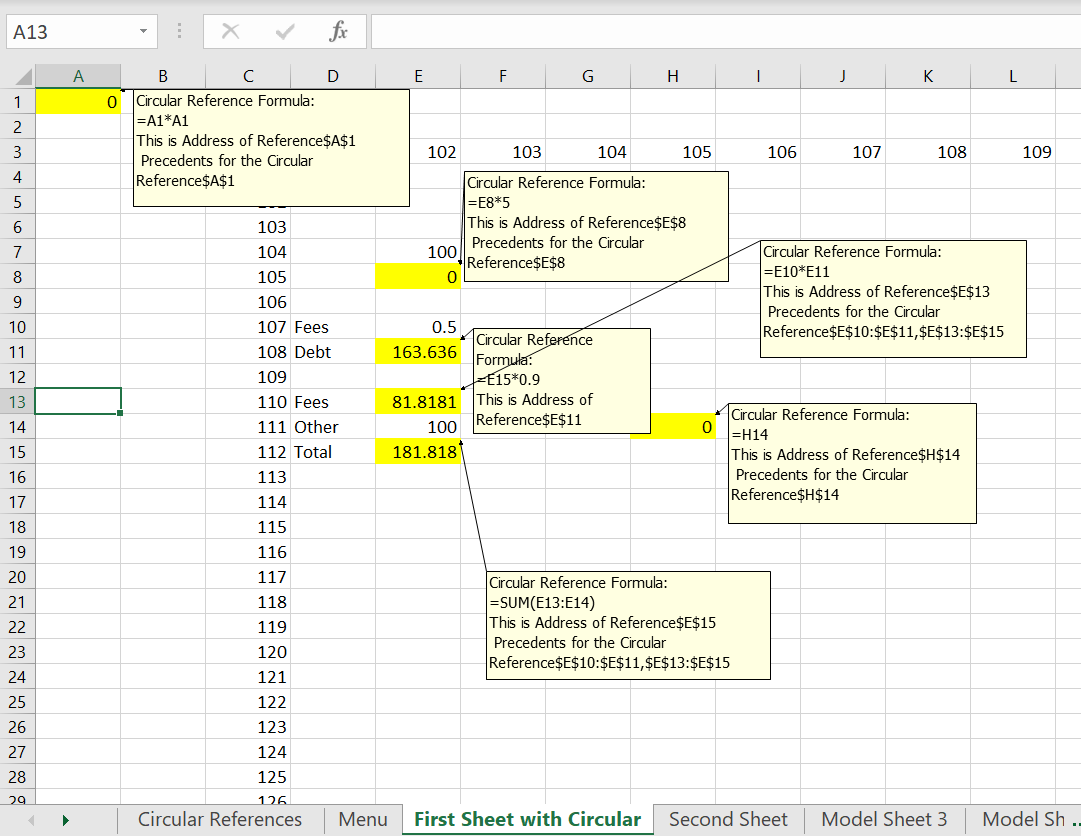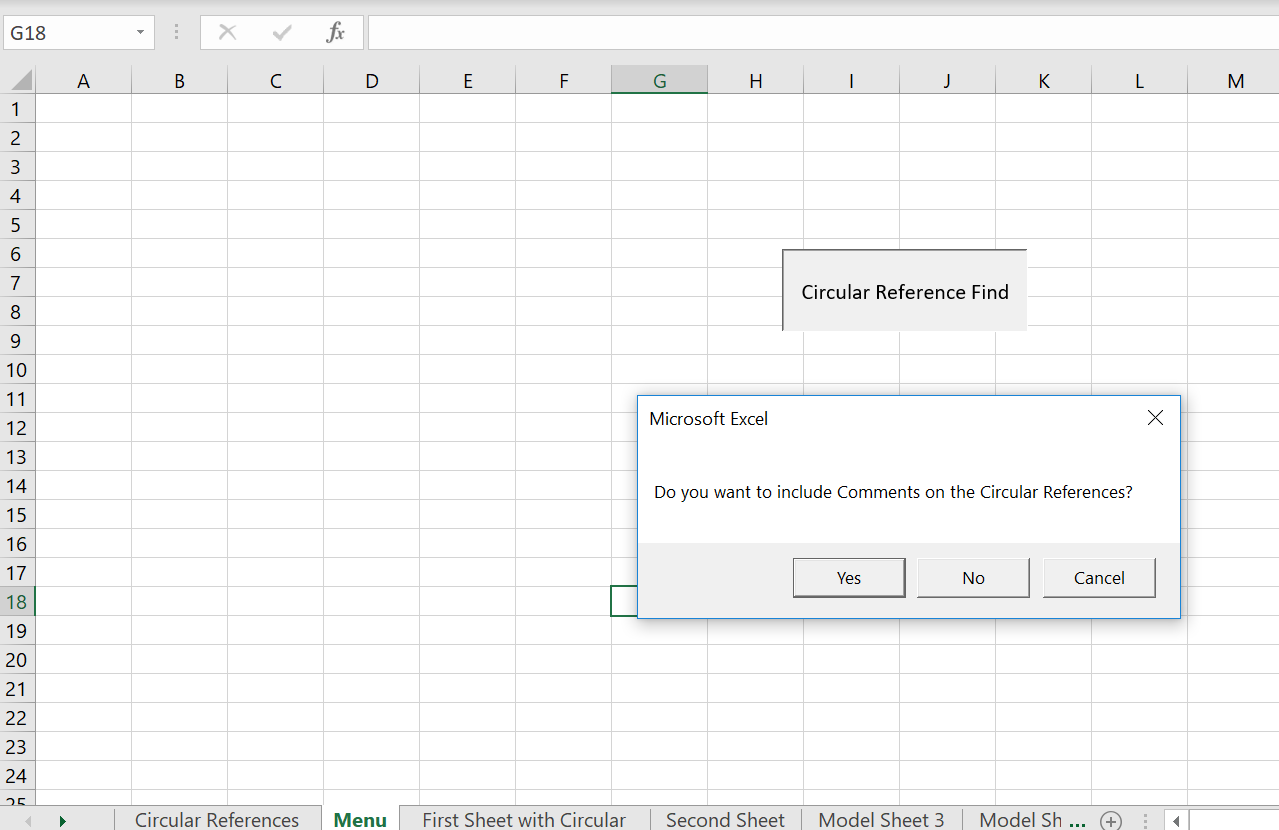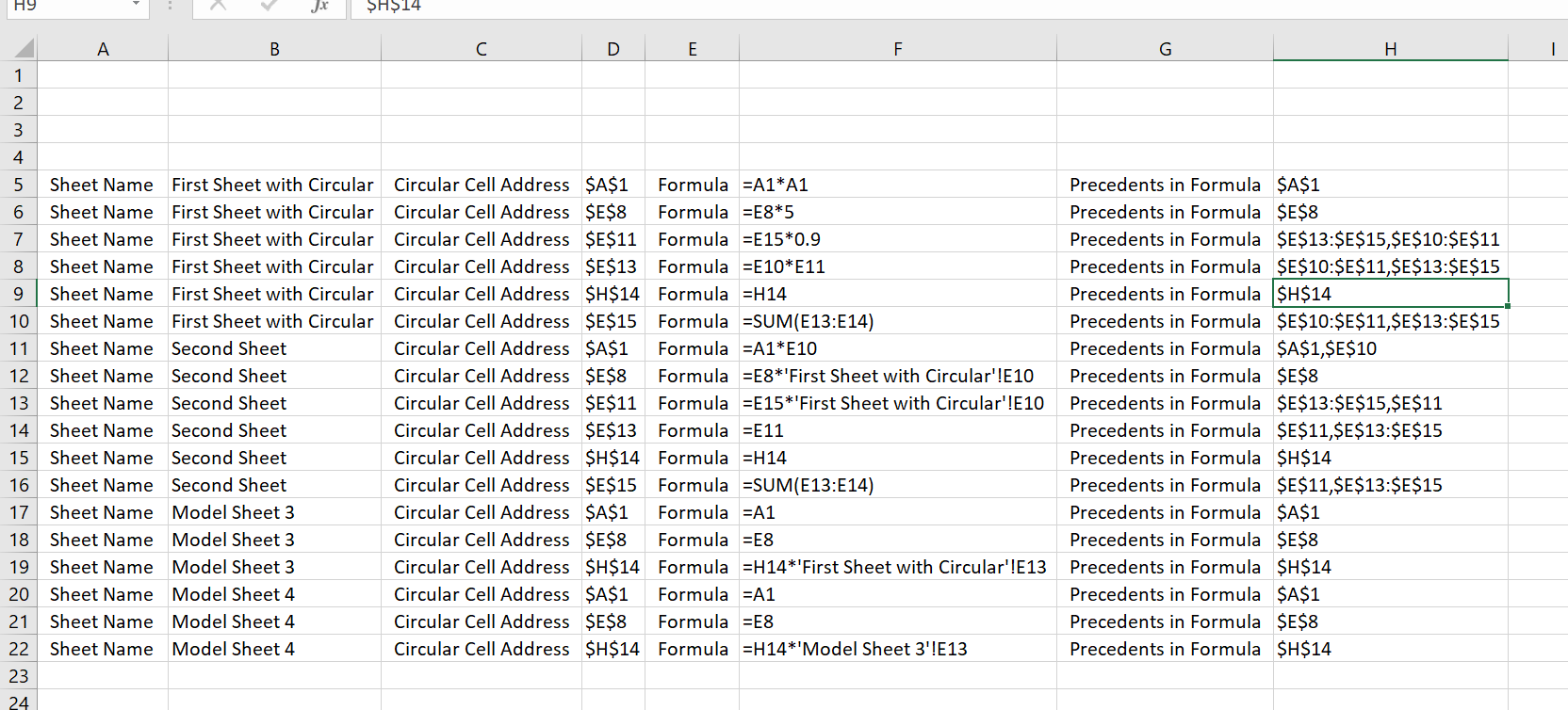# VBA Explanations

This page documents and explains some of the VBA code used in the generic macros file. I walk through the fundamental use of CELLS and FOR NEXT which is the foundation of many of the methods used in the file. My style in programming is very old fashioned and different from other techniques you will find on the internet. The methods for constructing macros that copy to the right and that create colours are explained along with some of the UDF’s in the generic macros file. You can find all of the VBA code in the generic macros file and in the read PDF file by just going to the macro list.

The buttons below allow you to download the Generic Macro and Read PDF to Excel files.  After you open the files, press ALT, F8 and then you can look at the VBA code.## Error Trapping with VBA

The file and the VBA code that you can download below illustrates the issue of error trapping.  It took me many years to figure out the problem of where you have an error trap in a loop.  Unless you get out of the entire loop you will have problems.

## VBA Illustration Using Circular Reference Find

In demonstrating VBA code, there are a bunch of examples where you find a cell and do something with that cell.  It could be finding a cell that has a precedent from another file and colouring that cell.  It could be finding a cell with the sheet name that you want to delete. It could be finding a cell so you can copy to the right. It could be finding numbers.

I have made a video that walks through the VBA code and some VBA principles.  It is intended to be the second in a series of videos the describe VBA code.  The first video describes how to make a data table where the goal seek is operated for each sensitivity case. I use the code in the circular reference find macro to illustrate a few principles that include:

• Use of msgbox
• Use of inputbox
• Finding circular references
• Error Trapping in Loops
• For/Next Loops
• VBA philosophy of Workbooks, Sheets and Cells
• Using Recorded Macros

The VBA Code finds, colours and lists circular references.  The file includes various different circular references as illustrated in the screenshot below.  The coloured cells contain circular references and the colouring is accomplished with the VBA code.  In addition, the VBA code includes the option to add comments to the circular references which are created by the code.  The process of adding comments and adding colours is part of the video description.When operating the VBA code, a few message boxes and input boxes are used for options.  A message box is used for a YES/NO question involving whether to add comments.  Input boxes are used to select which sheets are used for adding colours and comments to the all of the circular references.  The screenshot below illustrates theThe VBA code adds a sheet and lists the address and the formulas for the circular references. The VBA code uses the CELLS statement with a loop to do this.  Results of this process are illustrated in the screenshot below and discussed in detail in the video.The VBA code that is used to find the circular references is listed below.

.

```Sub Find_circular()

' Before Loop Around Sheets
MsgBox " This progam finds circular references and lists them on a sheet"
comment_question = MsgBox("Do you want to include Comments on the Circular References?", vbYesNoCancel) ' 6 is yes and 7 is no
'
' Input box
'
Dim start_sheet, end_sheet As Single
start_sheet = InputBox("Number (not name) of Starting Sheet")
end_sheet = InputBox("Number (not name) of Final Sheet")
'
' Cells, Sheets and Workbooks Add a sheet
'
Application.DisplayAlerts = False ' You are going to delete a sheet and you don't want the are you sure question
On Error Resume Next ' Error trapping can be a real pain

Sheets("Circular References").Delete ' May or may not exist
ActiveSheet.Name = "Circular References" ' Re-name the sheet and understand that you have workbooks, sheets, and cells

Count_of_circular_references = 4 ' Initialsise row number for output. I do not bother defining it
'
' Loop around all of the sheets
'
Dim cell_string1 As String
For Sheet = start_sheet To end_sheet ' For loop and other kinds of loops are key in VBA
Sheets(Sheet).Select ' Sheets() with name or number
base_sheet = ActiveSheet.Name

Cells.Select ' Select all of the cells
Selection.ClearFormats ' Clear all of the comments from the sheet
ActiveSheet.Calculate

For Row = 1 To 20 ' Get used to looping around rows and columns
For col = 1 To 20

cell_string = Cells(Row, col).Formula ' Can get formula and address from a cell

cell_string1 = "'" & cell_string ' So you can print out a formula

If Left(cell_string, 1) = "=" Then
On Error GoTo notcircular ' BIG point. When trap error, need to get out of the loop
'
' This is the big formula to find if there are circular references
' If cell intersects with precedents, cell has circular reference.
'

Count_of_circular_references = Count_of_circular_references + 1 ' count circular references in sheet

Sheets("Circular References").Cells(Count_of_circular_references, 3) = " Circular Cell Address "
Sheets("Circular References").Cells(Count_of_circular_references, 5) = " Formula "
Sheets("Circular References").Cells(Count_of_circular_references, 6) = cell_string1
Sheets("Circular References").Cells(Count_of_circular_references, 7) = " Precedents in Formula "
Sheets("Circular References").Cells(Count_of_circular_references, 8) = cell_precedent
Sheets("Circular References").Cells(Count_of_circular_references, 1) = " Sheet Name "
Sheets("Circular References").Cells(Count_of_circular_references, 2) = base_sheet

Cells(Row, col).Select ' Select the cells for colouring

With Selection.Interior
.Pattern = xlSolid
.PatternColorIndex = xlAutomatic
.Color = 65535
End With

If comment_question = 6 Then

Selection.Comment.Visible = True
Selection.Comment.Text Text:="Circular Reference Formula:" & cell_string & Chr(10) & "Address" & cell_address & Chr(10) & _
" Precedents" & cell_precedent

End If
End If

Next col
skipitem:

Next Row

Next Sheet

Sheets("Circular References").Select

Columns("A:H").Select
Columns("A:H").EntireColumn.AutoFit

Exit Sub

notcircular:

Resume skipitem:

End Sub```

.

## Technical Discussion of Colouring Macros

A lot of the excel short-cuts are pretty useless and you can use the menu or the mouse just as fast. But there are a few short-cuts that can be helpful are the subject of this page.

When you press CNTL, ALT, C if the generic macros file is open somewhere